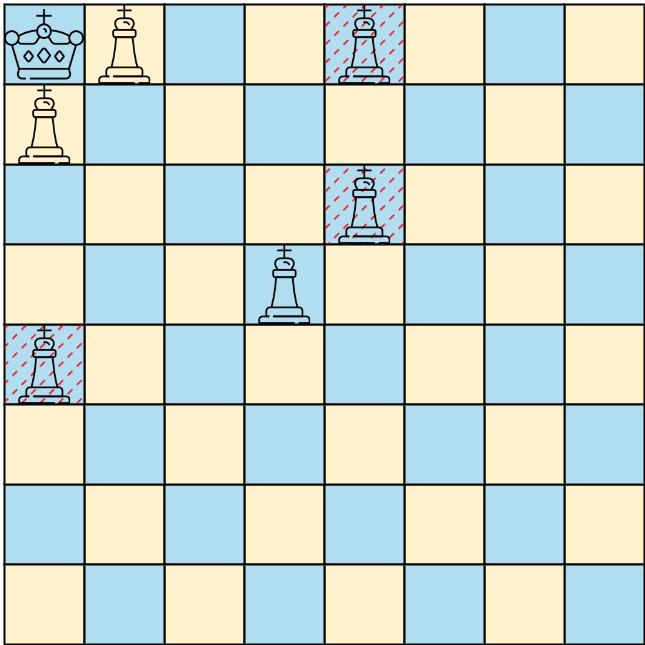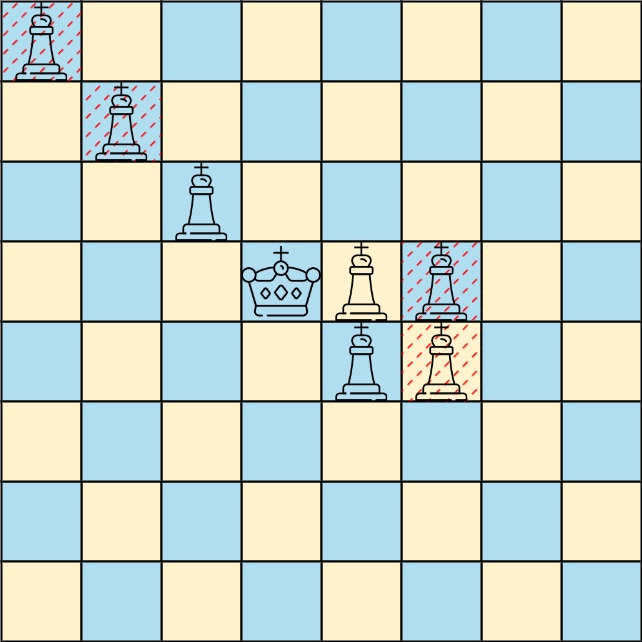## Algorithm

Problem Name: 1222. Queens That Can Attack the King

On a 0-indexed `8 x 8` chessboard, there can be multiple black queens ad one white king.

You are given a 2D integer array `queens` where `queens[i] = [xQueeni, yQueeni]` represents the position of the `ith` black queen on the chessboard. You are also given an integer array `king` of length `2` where `king = [xKing, yKing]` represents the position of the white king.

Return the coordinates of the black queens that can directly attack the king. You may return the answer in any order.

Example 1:```Input: queens = [[0,1],[1,0],[4,0],[0,4],[3,3],[2,4]], king = [0,0]
Output: [[0,1],[1,0],[3,3]]
Explanation: The diagram above shows the three queens that can directly attack the king and the three queens that cannot attack the king (i.e., marked with red dashes).
```

Example 2:```Input: queens = [[0,0],[1,1],[2,2],[3,4],[3,5],[4,4],[4,5]], king = [3,3]
Output: [[2,2],[3,4],[4,4]]
Explanation: The diagram above shows the three queens that can directly attack the king and the three queens that cannot attack the king (i.e., marked with red dashes).
```

Constraints:

• `1 <= queens.length < 64`
• `queens[i].length == king.length == 2`
• `0 <= xQueeni, yQueeni, xKing, yKing < 8`
• All the given positions are unique.

## Code Examples

### #1 Code Example with Python Programming

```Code - Python Programming```

``````
class Solution:
def queensAttacktheKing(self, queens: List[List[int]], king: List[int]) -> List[List[int]]:
def dfs(dr, dc, r, c):
while 0 <= r <= 7 and 0 <= c <= 7:
if (r, c) in q:
res.append([r, c])
break
r += dr
c += dc
q = set((r,c) for r, c in queens)
res = []
for dr, dc in (-1, -1), (-1, 0), (-1, 1), (0, 1), (1, 1), (1, 0), (1, -1), (0, -1):
dfs(dr, dc, *king)
return res
``````
Copy The Code &

Input

cmd
queens = [[0,1],[1,0],[4,0],[0,4],[3,3],[2,4]], king = [0,0]

Output

cmd
[[0,1],[1,0],[3,3]]

### #2 Code Example with C# Programming

```Code - C# Programming```

``````
using System.Collections.Generic;
using System.Linq;

namespace LeetCode
{
public class _1222_QueensThatCanAttackTheKing
{
public IList> QueensAttacktheKing(int[][] queens, int[] king)
{
var result = new List>();
var set = queens.Select(q => (q, q)).ToHashSet();

for (int r = -1; r <= 1; r++)
for (int c = -1; c <= 1; c++)
for (int length = 1; length < 8; length++)
{
var qc = king + c * length;
var qr = king + r * length;
if (qc < 0 || qc >= 8 || qr < 0 || qr >= 8) break;
if (set.Contains((qr, qc)))
{
result.Add(new int[] { qr, qc });
break;
}
}

return result;
}
}
}
``````
Copy The Code &

Input

cmd
queens = [[0,1],[1,0],[4,0],[0,4],[3,3],[2,4]], king = [0,0]

Output

cmd
[[0,1],[1,0],[3,3]]